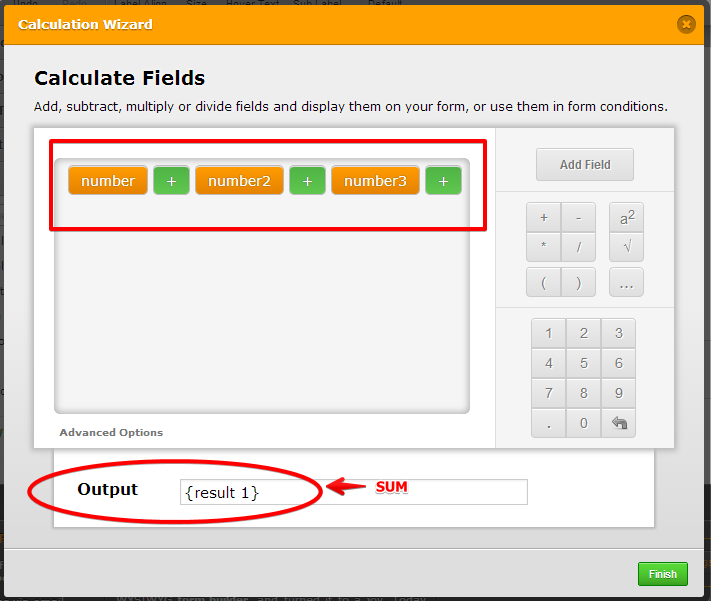# Calculate field option and Sum function in calculation wizard

•businet
Asked on May 17, 2014 at 10:33 PM
I am tryiing to use a similar feature but can not see the Calculate Filed option. I have cleared the form cache and browser cache and can not see it. Is it still available? It would be great if you guys introduced a 'Sum' function in the calculation wizard too. Any help will be appreciated, thanks.
•Welvin
Answered on May 18, 2014 at 06:41 AM

Hi,

The calculation feature is moved as a Widget to our widgets section. Please check this guide: https://www.jotform.com/help/259-How-to-perform-calculation-in-the-form

Let me also add that if you have a field, for example, a dropdown field, each option can have a calculation values. You just need to set it into the field properties. Please check this screenshot:

Settings > Show Properties > Calculate Values:This feature in each field is useful if you want to hide the prices and just show the text options.

Also check this related guides for calculation:

https://www.jotform.com/help/267-Form-Calculation-Math-Function-Reference

https://www.jotform.com/help/268-How-to-Insert-Text-or-Mathematical-Caculation-into-a-field-using-a-Condition

https://www.jotform.com/help/270-How-to-Pass-a-Calculated-Value-in-a-Paypal-Field-of-a-Second-Form

Hope that helps. Let us know if you have any further questions.

Thanks

•Welvin
Answered on May 18, 2014 at 11:06 AM

Thanks

•businet
Answered on May 18, 2014 at 03:36 PM

Thanks but all I am looking for is a simple 'Sum' function. I do not think it is in the list?

•jonathan
Answered on May 18, 2014 at 06:02 PM

@businet

Hi, you meant the "Sum function" was not available in the Math Function Reference right?

To have SUM() using the Calculation widget, you just use addition function.Basic math operators like (add, multiply, division and subtraction) were already available. I think most of those listed in the math reference were advance math functions instead.

Hope this help. Please inform us if you have further question.

Thanks!

•businet
Answered on May 18, 2014 at 06:07 PM

Thanks I have used this function. But I have 18 different questions. It would have been easier if I just put in 'sum(Q1.Q18)'. The Sum function is a standard mathematical function available say in Excel but not the JotForm Math Function Reference. i just find this strange when there are many other, often not used, functions there.

•BryanPaulo
Answered on May 18, 2014 at 06:29 PM

Hello businet,

The Sum function of excel won't work perfectly for forms unlike spreadsheets. Spreadsheet cells has a constant cell address which you can reference to sum up their values. Forms on the other hand are fields of different types and their labels vary from time to time.

Should you wish to use a spreadsheet to calculate values, you may wanna consider using the Spreadsheet widget which can use formulas to calculate the values.

Bryan